Home | | Chemistry 11th std | Brief questions and answers: Chemistry: Chemical bonding

# Brief questions and answers: Chemistry: Chemical bonding

Brief questions and answers, Answer the following questions : Chemistry: Chemical bonding

Chemical bonding | Chemistry

31. Define the following

i. Bond order

ii. Hybridisation

iii. σ- bond

Bond order :

Bond order gives the number of covalent bonds berween the two combining atoms

Bond order = [ Nb – Na ] / 2

Hybridisation :

Hybridisation is the process of mixing of atomic orbitals of the same atom with comparable energy to form equal number of new equivalent orbitals with same energy.

σ - bend :

When two atomic orbitals overlap linearly along the axis, the resultant bond is called a sigma (σ) bond. This overlap is also called ‘head -on everlap’ or ‘axial overlap’.

32. What is a pi bond?

When two atomic orbitals overlaps sideways, the resultant covalent bond is called a pi (π) bond.

33. In CH4, NH3 and H2O, the central atom undergoes sp3 hybridisation - yet their bond angles are different. why?

i) MethaneTetrahedral (AB4 type)

No lone pair of electrons

Hence, bond angle is 109°28' (or) 109.5°

ii) Ammoniapyramidal shape (AB3L type)

Number of lone pairs = 1

Due to lp - bp repulsion, bond angle decreases to 107° 18’

iii) water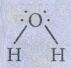Bent (V-shaped) - AB2L2 type.

Number of lone pairs = 2

Due to lp-bp repulsion, bond angle decreases to 104° 35’

34. Explain Sp2 hybridisation in BF3

The electronic configuration of Boron● In the GS, Boron has only one unpaired electron in the valence shell.

● Three unpaired e s are required to form three covalent bonds with fluorine atoms.

● So, one electron from the 2s orbital is promoted to the 2p orbital in the excited state.

● Hence, three equivalent sp2 hybridised orbitals are generated.

● This three sp2 hybridised orbitals of Boron now overlap with the 2p orbitals of three Fluorine atoms.

● This overlap takes place along the axis and forming three covalent bonds.35. Draw the M.O diagram for oxygen molecule calculate its bond order and show that O2 is paramagnetic.● Bond order = [ Nb – Na ] / 2 = [10 – 6] / 2 = 2

● Oxygen molecule has two unpaired electrons. Hence, it is paramagnetic.

36. Draw MO diagram of CO and calculate its bond order.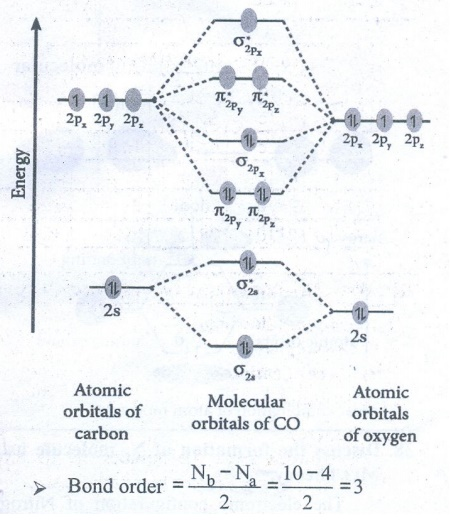● Bond order = [ Nb – Na ] / 2 = [10–4] / 2 = 3

37. What do you understand by Linear combination of atomic orbitals in MO theory.

There are two interactions of Linear combination of atomic orbitals (LCAO)

a) Constructive interaction

As a result of constructive interference of atomic orbitals, Bonding molecular orbitals are formed.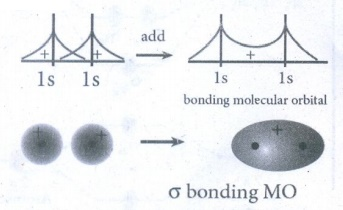b) Destructive interaction

As a result of destructive interference of atomic orbitals, Anti bonding molecular orbitals are formed.Linear combination of atom molecules

38. Discuss the formation of N2 molecule using MO Theory

The electronic configuration of Nitrogen atom 1s2 2s2 sp3

The electronic configuration of Nitrogen molecule (σ1s )2 (σ*1s )2 2s )2 (σ*2s )2 2py )2 2pz )2 2px )2● Bond order = [ Nb – Na ] / 2 = [10 – 4] / 2 = 3

● N2 molecule has no unpaired electrons. Hence, it is diamagnetic.

39. What is dipolment?

The polarity of a covalent bond can be measured in terms of dipole moment.

μ = q × 2d

μ is a vector scale. The direction of μ from the negative charge to positive charge. Unit of Dipolement is columb meter (Cm).

40. Linear form of carbondioxide molecule has two polar bonds. yet the molecule has Zero dipolement why?

● Linear form of CO2 has two polar bonds.

● The dipole moments of two polar bonds are equal in magnitude but have opposite direction.● The net dipole moment of CO2 is = 0

That is,41. Draw the Lewis structures for the following species.

i) NO3 ii) SO42– iii) HNO3 iv) O342. Explain the bond formation in BeCl2 and MgCl2.

Formation of BeCl2:

Electronic configuration of Be (Z = 4) in● Each of the sp hybridised orbitals linearly overlap with orbital of the chlorine to form a covalent bond between Be and Cl.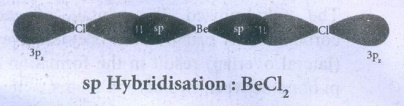Formation of MgCl2

● Mg (z = 12) loses two es and forming a stable configuration of Ne.

Mg           →         Mg2+     +        2e-

(2,8,2)                   (2,8)

● Two Cl(z = 17) accepts two es (one per each) and forming a stable configuration of Ar.

2Cl      +    2e−      →      2Cl

(2,8,7)                           (2,8,8)

● One Mg2+ and two Cl ions are held together by electrostatic attractive force.

43. Which bond is stronger σ or π? Why?

● σ - bond is stronger than the π-bond.

● The strength of a covalent bond depends upon the extent of overlap of atomic orbitals.

● Greater the overlap, larger is the energy released and stronger will be the bond formed.

● Overlaping along the axis (σ bond) is greater than the sidewise overlaping (π -bond).

44. Define bond energy.

The bond energy is defined as the minimum amount of energy required to break one mole of a particular bond in molecule in their gaseous state. The unit of bond energy is kJmol−1.

45. Hydrogen gas is diatomic where as inert gases are monoatomic – explain on the basis of MO theory.

● According to MO theory,

Bond order = [ Nb – Na ] / 2

Nb - total number of es in bonding molecular orbitals.

Na - total number of es in Antibonding molecular orbitals.

● Bond order gives the number of covalent bonds between the two combining atoms.

● From the MO diagram of H2 molecule,

Nb = 2, Na = 0

Therefore, Bond order = 1

H - H

Diatomic

● From the MO diagram of Helium molecule, Nb = Na = 2

Therefore, Bond order = 0; He2 is not formed.

So, He like inert gases are monoatomic.

46. What is Polar Covalent bond? explain with example.

In the case of covalent bond formed between atoms having different electronegativities, the atom with higher electronegativity will have the greater tendency to attract the shared pair of electrons more towards itself than the other atom.

This leads to partial negative charge on the atom and partial positive charge on the another atom. This is called polar covalent bond.

Example: HF molecule Hδ+ − F δ-

(Electronegativities of Hydrogen and Fluorine are 2.1 and 4.0 respectively)

47. Considering x- axis as molecular axis, which out of the following will form a sigma bond.

i) 1s and 2py ii) 2Px and 2Px

iii) 2px and 2pz  iv) 1s and 2pz

i) 1s and 2py form a σ-bond

Ex: H - C bond is CH4 molecule

ii) 2PX and 2PX form a σ-bond

Ex: B - B bond in B2 molecule

iii) 2PX and 2PZ form a π-bond

Ex: B-F bond in BF3 molecule

iv) 1s and 2PZ form a σ-bond

Ex: H - N bond in NH3 molecule.

48. Explain resonance with reference to carbonate ion?

Three possible resonance structures can be drawn for carbonate ion (CO32−).● In this structures, the relative position of the atoms are same.

● They only differ in the position of bonding and lone pair of electrons.

● Such structures are called resonance structures and this phenomenon is called resonance.

49. Explain the bond formation in ethylene and acetylene.

Bond formation in Ethylene.

● Molecular fomula of Ethylene is : C2H4

● Electronic configuration of Carbon

In Ground state : 1s2 2s2 2px1 2py1 2pz0To satisfy the valency of carbon, promote an electron from 2s orbital to 2pz orbital.

In excited state : 1s2 2s1 2px1 2py1 2pz1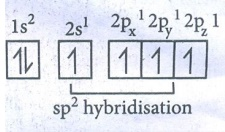In Hybridised state : sp2 sp2 sp2 2pz1● The three equivalent sp2 hybridised orbitals lying in the xy plane at an angle of 1200 to each other.

● The unhybridised 2pz orbital lies perpendicular to the xy plane.

● One of the sp2 hybridised orbitals of each carbon linearly overlaps with each other resulting the formation of C - C sigma bond.

● Other two sp2 hybridised orbitals of both carbons linearly everlap with the four 1s orbitals of four H atoms leading the formation of four C - H sigma bonds on each carbon.

● The unhybridised 2pz orbitals of each corbon atoms can overlap only sideways (lateral overlap) result in the formation of pi bond between two carbon atoms.Bond formation in Acetylene:

● Molecular formula of Acetylene is C2H2

● Electronic configuration of carbon

In ground state :1s2 2s2 2px1 2py1 2pz0To satisfy the valency of carbon, promote an electron from 2s orbital to 2pz orbital.

In excited state : 1s2 2s1 2px1 2py1 2pz1In Hybridised state : sp sp 2py1 2pz1● The 2s and 2px orbitals forming two equivalent sp hybridised orbitals.

● The unhybridised 2py and 2pz orbitals lie perpendicular to the molecular axis.

● One of the two sp hybridized orbitals of each carbon linearly overlap with each other, resulting the formation of C - C sigma bond.

● The other sp hybridised orbital of both carbon linearly overlap with two 1s orbitals of 2H atoms leading the formation of one C-H sigma bond on each carbon.

● The unhybridised 2Py and 2PZ orbitals of each Carbon atoms can overlap sideways (lateral overlap) result in the formation of pi bond between the two Carbon atoms.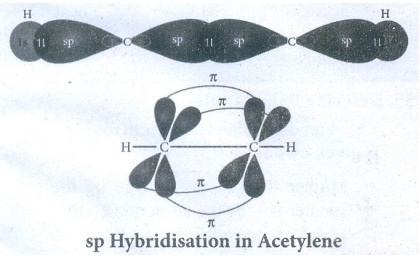50. What type of hybridisations are possible in the following geometeries?

a) octahedral

b) tetrahedral

c) square planer.

Geometry : Hybridisation

a) Octahedral : sp3d2

b) Tetrahedral : sp3

c) Square planar : sp2d

51. Explain VSEPR theory. Applying this theory to predict the shapes of IF7, and SF6

Shape of IF7 molecule:

● Valence shell electronic configuration of Iodine.● Iodine has 7sp3d3 hybridized orbitals.

● This orbitals linearly overlap with 2Pz orbitals of seven F atoms, leading the formation of 7 σ-bonds.

Geometry : Pentagonal bipyramidShape of SF6 molecule

● Valence shell electronic configuration of● Sulphur has 6 sp3d2 hybridised orbitals.

● This orbitals linearly overlap with 2Pz orbitals of six F atoms, leading the formation of 6 σ-bonds.

● Geometry : Octahedral.52. CO2 and H2O both are triatomic molecule but their dipole moment values are different. Why?

CO2 Geometry : Linear

● Eventhough CO2 has two polar bonds, Dipolemoment value is = 0

Reason: The DPM of two polar bonds are equal in magnitude but opposite in direction.H2O : Geometry : Bent molecule (V Shape)= 1.85 D

53. Which one of the following has highest bond order? N2, N+2 or N2

Bond order = [ Nb – Na ] / 2

Bond order of N2 = (10 – 4) / 2 = 3

Bond order of N2+ = (9 – 4) / 2 = 2.5

Bond order of N2 = (10 – 5) / 2 = 2.5

Hence, N2 molecule has the Highest bond order.

54. Explain the covalent character in ionic bond.

Ionic compounds show partial covalent character.

Ex: LiCl is an ionic compound. But, it shows covalent character. Therefore, it is soluble in organic solvent like Ethanol.

This can be explained on the basis of ‘Polarisation’.

● The positively charged cation attracts the valence electrons of anion while repelling the nucleus.

● This causes a distortion in the electron cloud of the anion and its electron density drifts towards the cation.

● This results in some sharing of the valence electrons between these ions.

● Thus, a partial covalent character is developed between them. This is called polarisation.

● The ability of a cation to polarise an anion is called ‘polarising ability’. The tendency of an anion to get polarized is called polarisability.

55. Describe fajan's rule.

The extend of polarisation in an ionic compound is given by Fajans rule.

● Higher the positive charge on the cation, greater will be the attraction on the electron cloud of the cation.

● Higher the magnitude of negative charge on the anion, greater is its polarisability.

● Hence, the increase in charge on cation or in anion, increases the covalent character.

Example:

Consider the ionic compounds: AlCl3, MgCl2 and NaCl.

Increasing order of cationic size

Na+ < Mg2+ < Al3+

● Order Covalent character :

Covalent character : LiCl > NaCl

Reason: size of Li+ < size of Na+

Li+ has the higher polarising power.

● Covalent character : Lil > LiCl

Reason: Size of I > size of Cl.

I will be more polarised.

Study Material, Lecturing Notes, Assignment, Reference, Wiki description explanation, brief detail
11th Chemistry : UNIT 10 : Chemical bonding : Brief questions and answers: Chemistry: Chemical bonding |